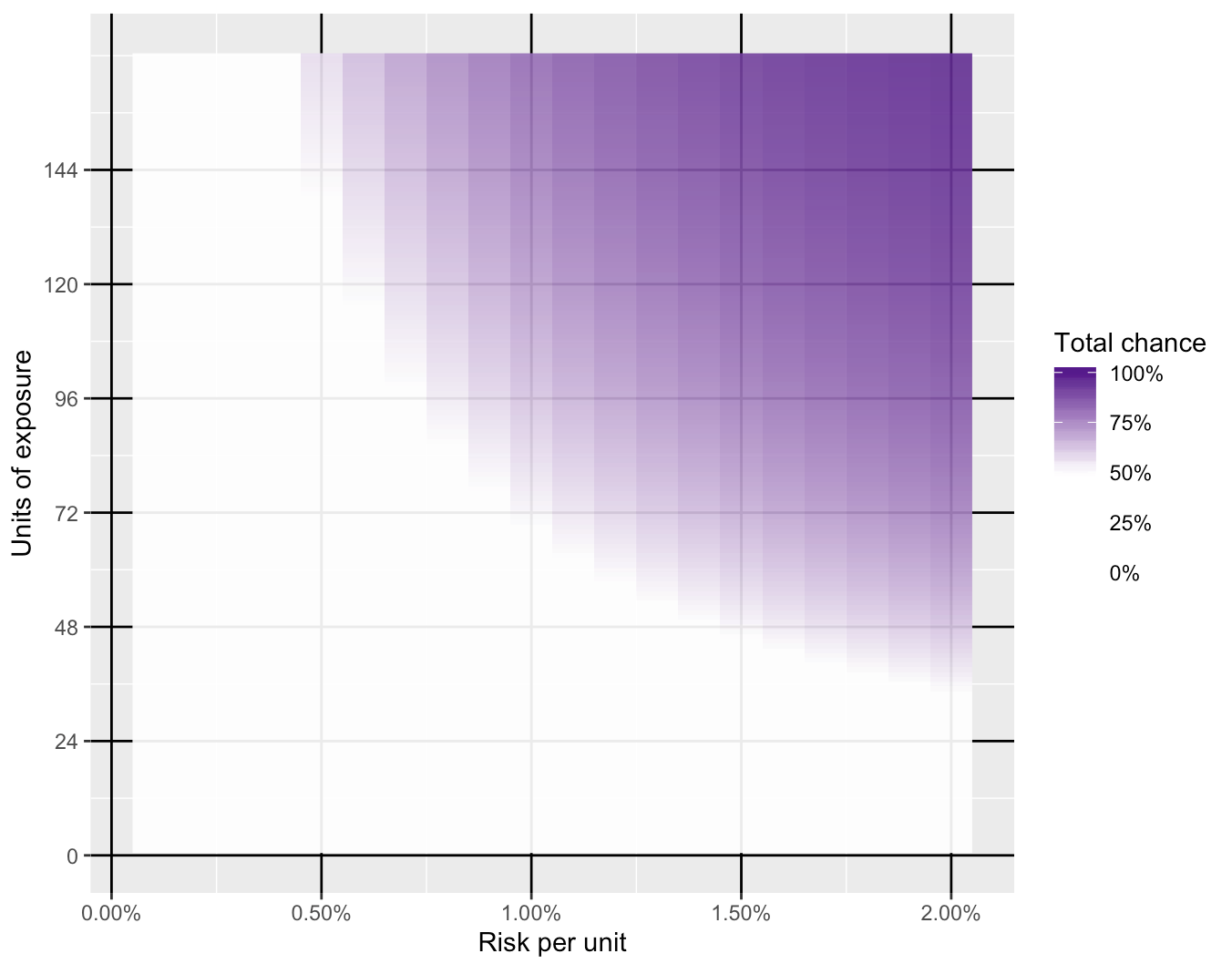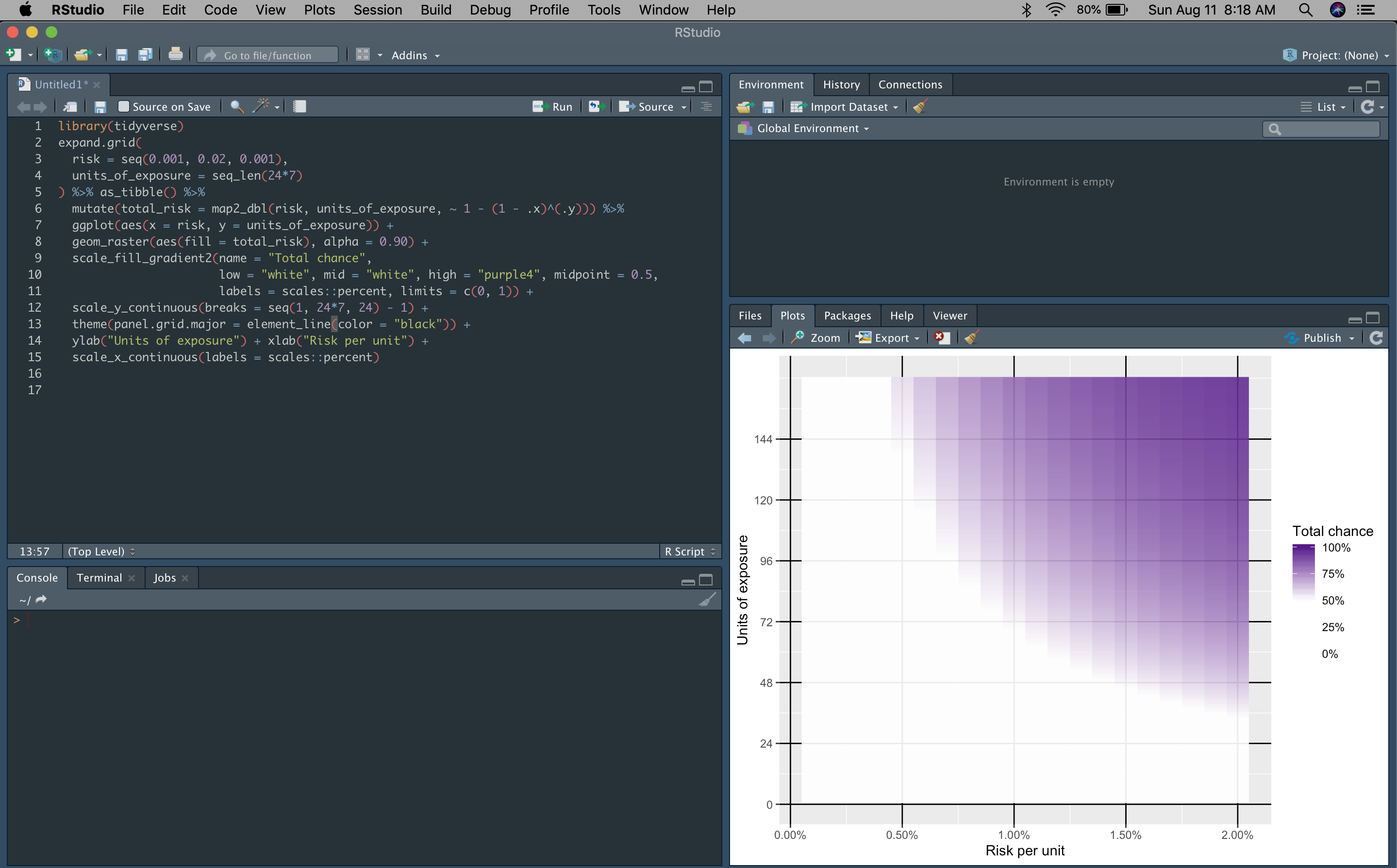Instantly share code, notes, and snippets.

#statwonk/risk_adds_up.R Last active Aug 11, 2019

Risk adds up. This code piece answers, "how quickly?" https://twitter.com/statwonk/status/1160542394544267265
 library(tidyverse) expand.grid( risk = seq(0.001, 0.02, 0.001), units_of_exposure = seq_len(24*7) ) %>% as_tibble() %>% mutate(total_risk = map2_dbl(risk, units_of_exposure, ~ 1 - (1 - .x)^(.y))) %>% ggplot(aes(x = risk, y = units_of_exposure)) + geom_raster(aes(fill = total_risk), alpha = 0.90) + scale_fill_gradient2(name = "Total chance", low = "white", mid = "white", high = "purple4", midpoint = 0.5, labels = scales::percent, limits = c(0, 1)) + scale_y_continuous(breaks = seq(1, 24*7, 24) - 1) + theme(panel.grid.major = element_line(color = "black")) + ylab("Units of exposure") + xlab("Risk per unit") + scale_x_continuous(labels = scales::percent)
Owner Author

### statwonk commented Aug 11, 2019 • edited

 The visualization shows that the risk in a given unit of time has a strong influence on how many units of exposure can be experienced before the event occur. It also shows how reduction of risk relates to the number of units of exposure we can take without experiencing the event.to join this conversation on GitHub. Already have an account? Sign in to comment
You can’t perform that action at this time.